# Macrorealism

MPQ theory wednesday seminar by Lucas Clemente

2015-01-14

This is joint work with Johannes.

The paper will be on the arxiv soonish :)

# Schrödinger's cat!What else?

# Macroscopic superpositions

Molecules, atomic gases, superconducting qubits, nanomechanics, …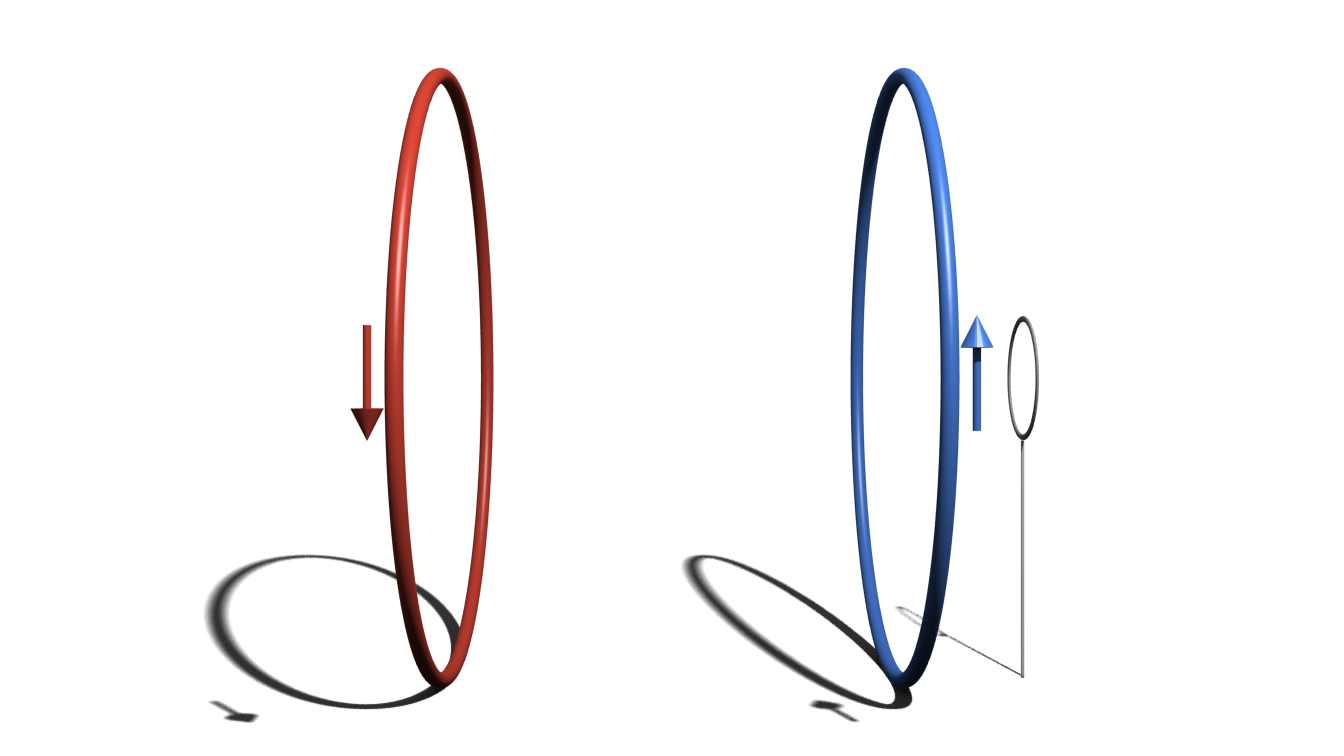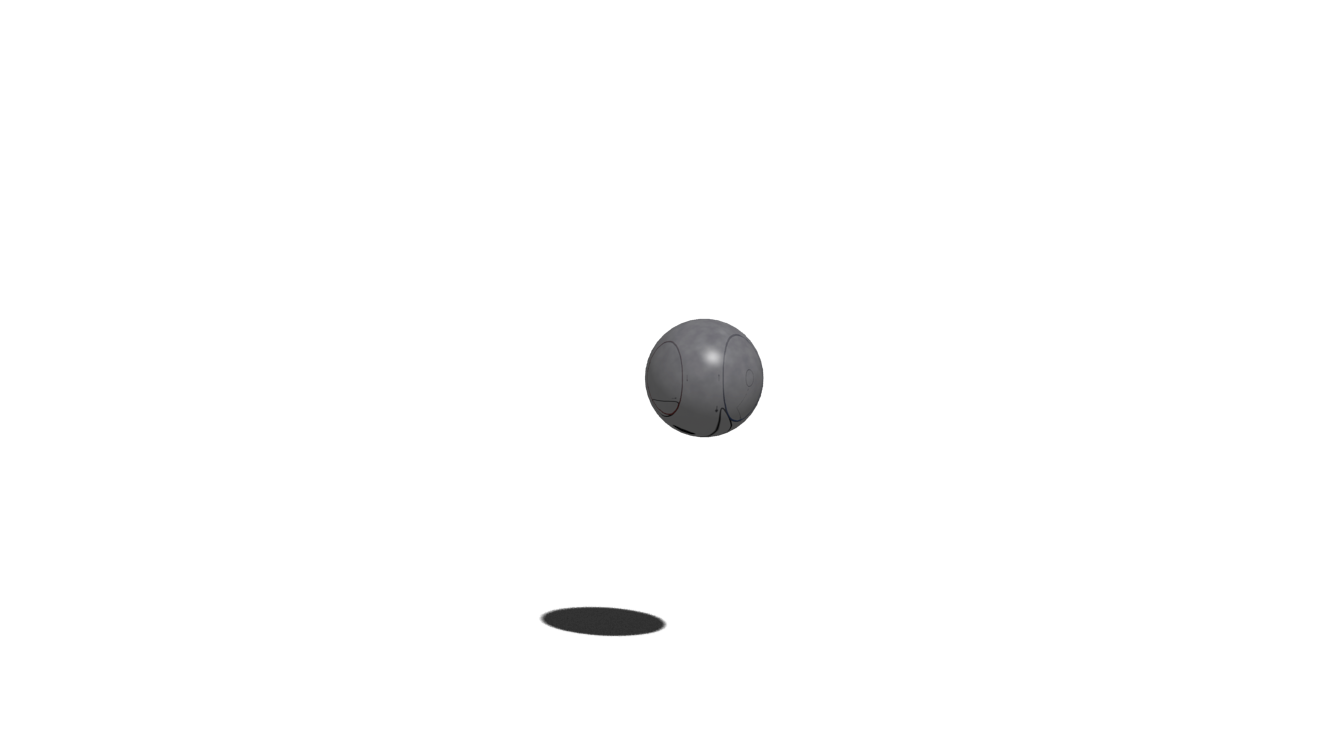# Macroscopic Realism

Legget, Garg 1985

The world view that macroscopic physical properties
are independent of measurements.

# 1. Macrorealismper se

A macroscopic object occupies
a definite macrostate at all times.

# 2. Noninvasive Measurements

It is possible to measure the state
without changing it (or the future evolution).

# Legget-Garg Inequalities

## A simple experiment

Macro-observable $Q = \pm 1$

Measurements at times $t_0, t_1, t_2$ with outcomes $Q_i$

Correlations $C_{ij} \equiv \langle Q_i Q_j\rangle = p_{++} + p_{--} - p_{+-} - p_{-+}$

# Joint Probability Distribution

$P(Q_0, Q_1, Q_2)$

at times $t_0$, $t_1$, $t_2$

$C_{01} = [P(+,+,+) + P(+,+,-)] \\+ [P(-,-,+) + P(-,-,-)] \\- [P(+,-,+) + P(+,-,-)] \\- [P(-,+,+) + P(-,+,-)]$ $C_{01} + C_{12} - C_{02} = 1 - 4[P(+,-,+) + P(-, +, -)]$

# Legget-Garg Inequality

$C_{01} + C_{12} - C_{02} \leq 1$

QM, qubit: $1.5$

QM, maximum: $3$

Budroni, Emary, PRL (2014)

# MR ⇒ LGI

## … but not the other way

LGI cannot detect every violation of MR, see later

# Joint Probability

$P(Q_0, Q_1, Q_2)$

## Let's call it

$P_{012}(Q_0, Q_1, Q_2)$

# Measurements matter!

$P_{12}(Q_1, Q_2) \neq P_{012}(Q_1, Q_2)$

# No-signaling in time

$P_{2}(Q_2) = P_{12}(Q_2) = \sum_{Q_1'} P_{12}(Q_1', Q_2)$

Kofler, Brukner, PRA (2013)

## More NSITs

$\text{NSIT}_{(0)1}:~P_{1} = P_{01}$ $\text{NSIT}_{(1)2}:~P_{2} = P_{12}$ $\text{NSIT}_{(0)2}:~P_{2} = P_{02}$ $\text{NSIT}_{0(1)2}:~P_{02} = P_{012}$ $\text{NSIT}_{(0)12}:~P_{12} = P_{012}$

## Trivial: Arrow of time

$\text{NSIT}_{0(1)}:~P_{0} = P_{01}$ $\text{NSIT}_{0(2)}:~P_{0} = P_{02}$ $\text{NSIT}_{1(2)}:~P_{1} = P_{12}$ $\text{NSIT}_{01(2)}:~P_{01} = P_{012}$# Revisiting LGI

$C_{01} + C_{12} - C_{02} \leq 1$ $C_{01} \rightarrow C_{01(2)}$ $C_{12} \rightarrow C_{(0)12}$ $C_{02} \rightarrow C_{0(1)2}$

# LGI from NSIT

$C_{01} + C_{12} - C_{02} \leq 1$ $\text{AoT}:~P_{01} = P_{012}$ $\text{NSIT}_{(0)12}:~P_{12} = P_{012}$ $\text{NSIT}_{0(1)2}:~P_{02} = P_{012}$

Maroney, Timpson (2014)

## We've only used 2,3joint probabilitites# NSITs⇕macrorealism012

NSIT(1)2 + NSIT0(1)2 + NSIT(0)12 + AoT ⇔ MR012

# Example

## qubit / Mach-ZehnderinterferometerMixed initial states $P(+) = q$.

## Mach-Zehnder Interferometer

 $R_1 = R_2 = \frac{1}{2}$ $R_1 = \frac{1}{4}, R_2 = \frac{3}{4}$ $\text{LGI}_{012}$ $1 + 3 \cos \varphi \geq 0$ $\text{NSIT}_{(1)2}$ $q = \frac{1}{2}$ or $\varphi = \frac{n\pi}{2}$ $q = \frac{1}{2}$ or $\varphi = \frac{n\pi}{2}$ $\text{NSIT}_{0(1)2}$ $\varphi = \frac{n\pi}{2}$ $\varphi = \frac{n\pi}{2}$ $\text{NSIT}_{(0)12}$

# What does NSITmean in practice?

## NSIT(0)T$P_{\hat B}(b) = \operatorname{tr}(\hat B_b \hat U_T \hat \rho_0 \hat U_T^\dagger \hat B_b^\dagger)$ $\bar P_{\hat B|\hat A}(b) = \int \text{d}a\,\operatorname{tr}(\hat B_b \hat U_T \hat A_a \hat \rho_0 \hat A_a^\dagger \hat U_T^\dagger \hat B_b^\dagger)$

# NSIT(0)0

$P_{\hat B}(b) - \bar P_{\hat B|\hat A}(b) = \int \text{d}a\,\operatorname{tr}([\hat A_a \hat B_b, \hat B_b \hat A_a] \hat \rho_0) = 0$ $\int \text{d}a\,[\hat A_a \hat B_b, \hat B_b \hat A_a] = 0$

## General NSIT(0)0

$\forall \rho_0: \text{NSIT}_{(0)0} \Leftrightarrow \int \text{d}a\,[\hat A_a \hat B_b, \hat B_b \hat A_a] = 0$

## For projectors

$\forall \rho_0: \text{NSIT}_{(0)0} \Leftrightarrow [\hat A_a, \hat B_b] = 0$

# NSIT(0)T

$P_{\hat B}(b) = \operatorname{tr}(\hat B_b \hat U_T \hat \rho_0 \hat U_T^\dagger \hat B_b^\dagger)$ $\bar P_{\hat B|\hat A}(b) = \int \text{d}a\,\operatorname{tr}(\hat B_b \hat U_T \hat A_a \hat \rho_0 \hat A_a^\dagger \hat U_T^\dagger \hat B_b^\dagger)$

define $\tilde B_b^T \equiv \hat U_T^\dagger \hat B_b \hat U_T$

## General NSIT(0)T

$\forall \rho_0: \text{NSIT}_{(0)T} \Leftrightarrow \int \text{d}a\,[\hat A_a \tilde B^T_b, \tilde B^T_b \hat A_a] = 0$

## For projectors

$\forall \rho_0: \text{NSIT}_{(0)T} \Leftrightarrow [\hat A_a, \tilde B^T_b] = 0$

# Reference operators

• Coarse-grained coherent states
• Coarse-grained Fock measurements
$V = \int \text{d}b \sqrt{P_{\hat B}(b) \bar P_{\hat B|\hat A}(b)} \in [0,1]$

Bhattacharyya 1943

# Coherent states

$\hat A_\alpha = |\alpha\rangle\langle\alpha|, \hat B_\beta = |\beta\rangle\langle\beta|$ $\hat \rho_0 = |\gamma\rangle\langle\gamma|$ $V(T = 0) = \frac{2 \sqrt 2}{3} \approx 0.943$## Coherent state bins

Overlap for different bin sizes

blue: bin border, orange: center of bin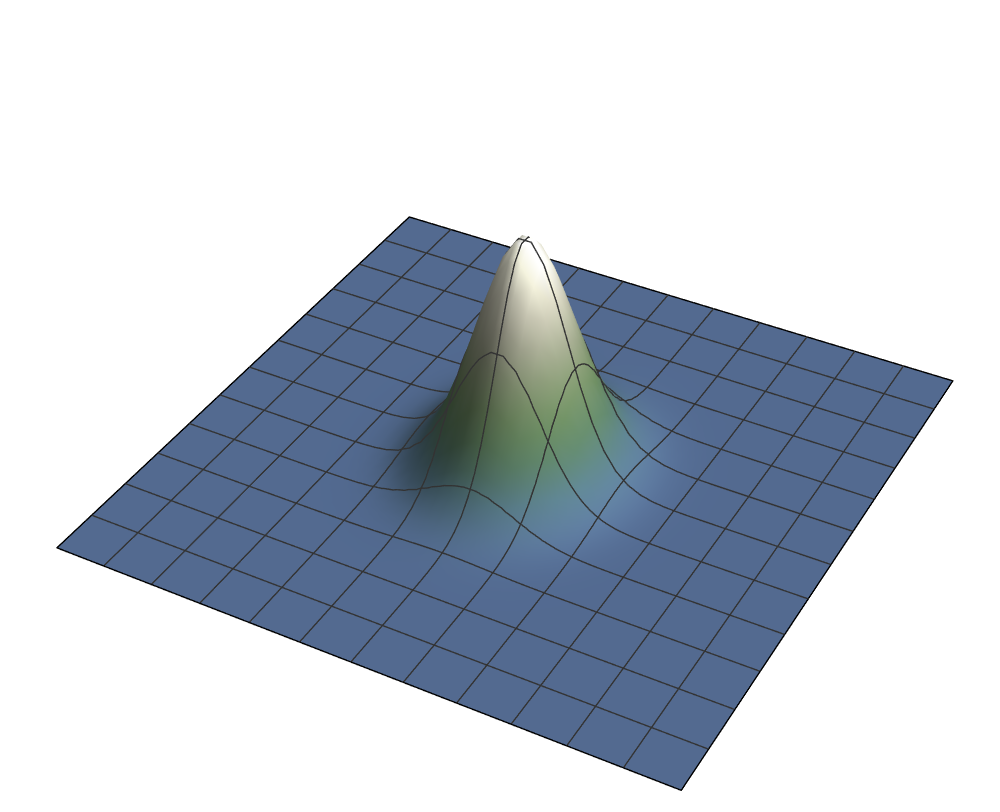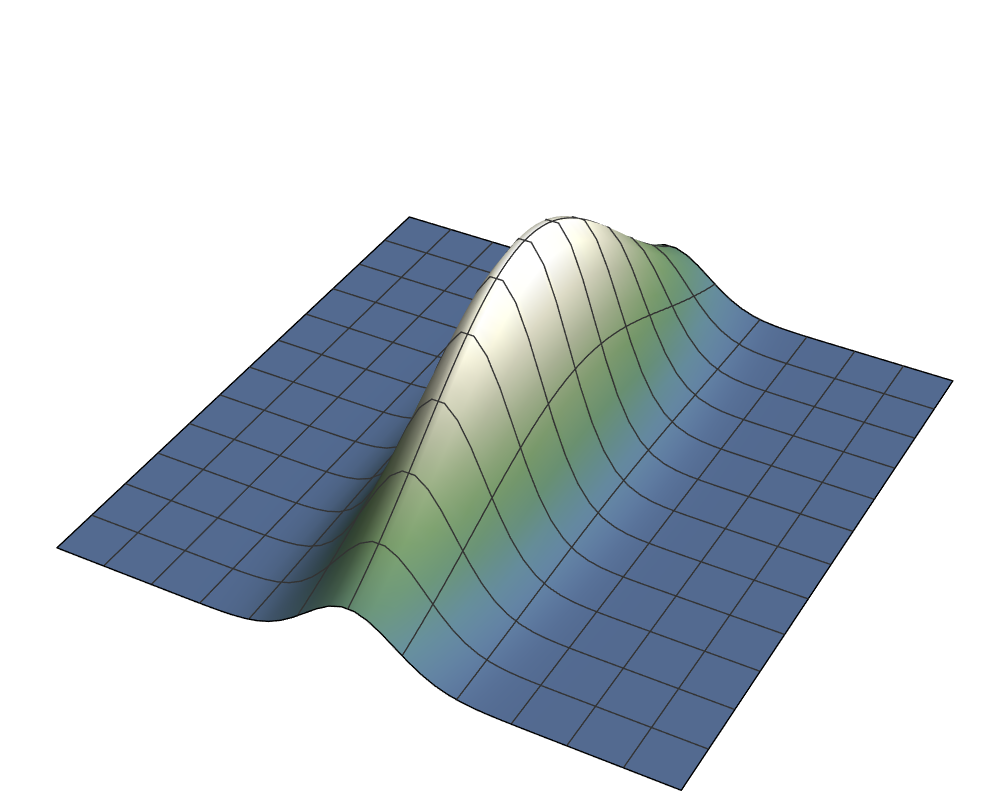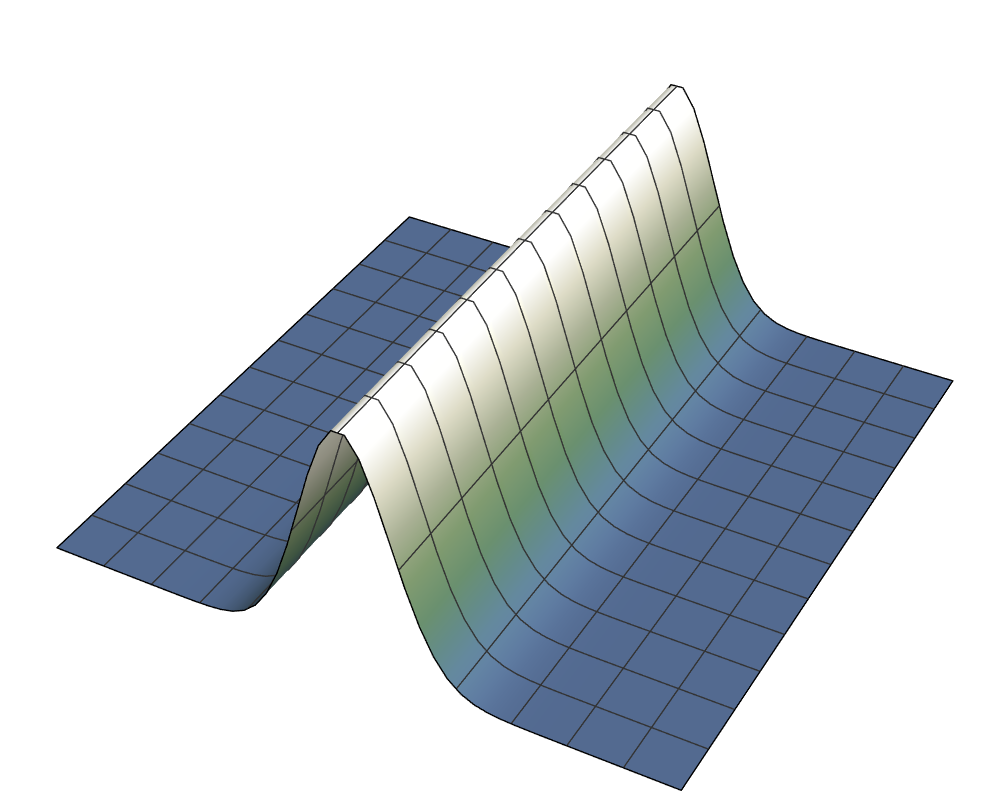$V = 1 ~~~~~~~~~~~~~~~~ V \approx 0.67 ~~~~~~~~~~~~~~~~ V \approx 0.17$

## Probability distributions for quadrature measurements

left to right: increase in sharpness